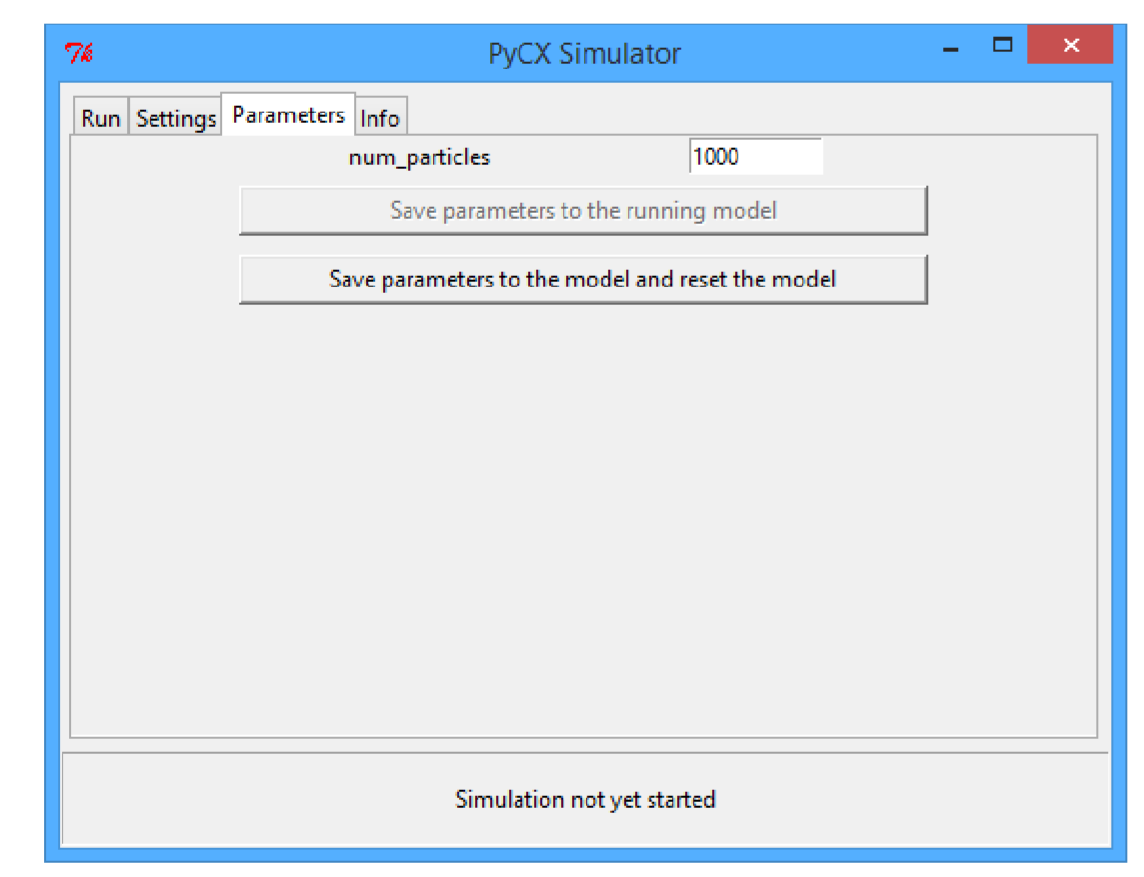$$\newcommand{\id}{\mathrm{id}}$$ $$\newcommand{\Span}{\mathrm{span}}$$ $$\newcommand{\kernel}{\mathrm{null}\,}$$ $$\newcommand{\range}{\mathrm{range}\,}$$ $$\newcommand{\RealPart}{\mathrm{Re}}$$ $$\newcommand{\ImaginaryPart}{\mathrm{Im}}$$ $$\newcommand{\Argument}{\mathrm{Arg}}$$ $$\newcommand{\norm}{\| #1 \|}$$ $$\newcommand{\inner}{\langle #1, #2 \rangle}$$ $$\newcommand{\Span}{\mathrm{span}}$$

# 10.4: Simulation without PyCX

$$\newcommand{\vecs}{\overset { \rightharpoonup} {\mathbf{#1}} }$$ $$\newcommand{\vecd}{\overset{-\!-\!\rightharpoonup}{\vphantom{a}\smash {#1}}}$$$$\newcommand{\id}{\mathrm{id}}$$ $$\newcommand{\Span}{\mathrm{span}}$$ $$\newcommand{\kernel}{\mathrm{null}\,}$$ $$\newcommand{\range}{\mathrm{range}\,}$$ $$\newcommand{\RealPart}{\mathrm{Re}}$$ $$\newcommand{\ImaginaryPart}{\mathrm{Im}}$$ $$\newcommand{\Argument}{\mathrm{Arg}}$$ $$\newcommand{\norm}{\| #1 \|}$$ $$\newcommand{\inner}{\langle #1, #2 \rangle}$$ $$\newcommand{\Span}{\mathrm{span}}$$ $$\newcommand{\id}{\mathrm{id}}$$ $$\newcommand{\Span}{\mathrm{span}}$$ $$\newcommand{\kernel}{\mathrm{null}\,}$$ $$\newcommand{\range}{\mathrm{range}\,}$$ $$\newcommand{\RealPart}{\mathrm{Re}}$$ $$\newcommand{\ImaginaryPart}{\mathrm{Im}}$$ $$\newcommand{\Argument}{\mathrm{Arg}}$$ $$\newcommand{\norm}{\| #1 \|}$$ $$\newcommand{\inner}{\langle #1, #2 \rangle}$$ $$\newcommand{\Span}{\mathrm{span}}$$

Finally, I would like to emphasize an important fact: The PyCX simulator ﬁle used in this chapter was used only for creating a GUI, while the core simulation model was still fully implemented in your own code. This means that your simulation model is completely independent of PyCX, and once the interactive exploration and model veriﬁcation is over, your simulator can “graduate” from PyCX and run on its own.

For example, here is a revised version of Code 10.6, which automatically generates a series of image ﬁles without using PyCX at all. You can generate an animated movie from the saved image ﬁles using, e.g., Windows Movie Maker. This is a nice example that illustrates the main purpose of PyCX—to serve as a stepping stone for students andFigure $$\PageIndex{1}$$: New parameter setter for the number of particles implemented in Code 10.10.

researchers in learning complex systems modeling and simulation, so that it eventually becomes unnecessary once they have acquired sufﬁcient programming skills.Exercise $$\PageIndex{1}$$

Develop your own interactive simulation code. Explore various features of the plotting functions and the PyCX simulator’s GUI. Then revise your code so that it automatically generates a series of image ﬁles without using PyCX. Finally, create an animated movie ﬁle using the image ﬁles generated by your own code. Enjoy!